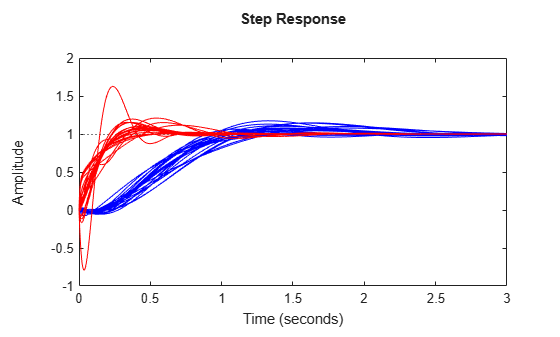# Robust Controller Design

This example shows how to design a feedback controller for a plant with uncertain parameters and uncertain model dynamics. The goals of the controller design are good steady-state tracking and disturbance-rejection properties.

Design a controller for the plant `G` described in Robust Controller Design. This plant is a first-order system with an uncertain time constant. The plant also has some uncertain dynamic deviations from first-order behavior beyond about 9 rad/s.

```bw = ureal('bw',5,'Percentage',10); Gnom = tf(1,[1/bw 1]); W = makeweight(.05,9,10); Delta = ultidyn('Delta',[1 1]); G = Gnom*(1+W*Delta)```
```G = Uncertain continuous-time state-space model with 1 outputs, 1 inputs, 2 states. The model uncertainty consists of the following blocks: Delta: Uncertain 1x1 LTI, peak gain = 1, 1 occurrences bw: Uncertain real, nominal = 5, variability = [-10,10]%, 1 occurrences Type "G.NominalValue" to see the nominal value, "get(G)" to see all properties, and "G.Uncertainty" to interact with the uncertain elements. ```

### Design Controller

Because of the nominal first-order behavior of the plant, choose a PI control architecture. For a desired closed-loop damping ratio ξ and natural frequency ${\omega }_{\mathit{n}}$, the design equations for the proportional and integral gains (based on the nominal open-loop time constant of 0.2) are:

`${K}_{p}=\frac{2\xi {\omega }_{n}}{5}-1,\phantom{\rule{0.2777777777777778em}{0ex}}{K}_{i}=\frac{{\omega }_{n}^{2}}{5}.$`

To study how the uncertainty in `G` affects the achievable closed-loop bandwidth, design two controllers, both achieving ξ = 0.707, but with different ${\omega }_{\mathit{n}}$ values, 3 and 7.5.

```xi = 0.707; wn1 = 3; wn2 = 7.5; Kp1 = 2*xi*wn1/5 - 1; Ki1 = (wn1^2)/5; C1 = tf([Kp1,Ki1],[1 0]); Kp2 = 2*xi*wn2/5 - 1; Ki2 = (wn2^2)/5; C2 = tf([Kp2,Ki2],[1 0]);```

### Examine Controller Performance

The nominal closed-loop bandwidth achieved by `C2` is in a region where `G` has significant model uncertainty. It is therefore expected that the model variations cause significant degradations in the closed-loop performance with that controller. To examine the performance, form the closed-loop systems and plot the step responses of samples of the resulting systems.

```T1 = feedback(G*C1,1); T2 = feedback(G*C2,1); tfinal = 3; step(T1,'b',T2,'r',tfinal)```The step responses for `T2` exhibit a faster rise time because `C2` sets a higher closed-loop bandwidth. However, as expected, the model variations have a greater impact.

You can use `robstab` to check the robustness of the stability of the closed-loop systems to model variations.

```opt = robOptions('Display','on'); stabmarg1 = robstab(T1,opt);```
```Computing peak... Percent completed: 100/100 System is robustly stable for the modeled uncertainty. -- It can tolerate up to 401% of the modeled uncertainty. -- There is a destabilizing perturbation amounting to 401% of the modeled uncertainty. -- This perturbation causes an instability at the frequency 3.74 rad/seconds. ```
`stabmarg2 = robstab(T2,opt);`
```Computing peak... Percent completed: 100/100 System is robustly stable for the modeled uncertainty. -- It can tolerate up to 125% of the modeled uncertainty. -- There is a destabilizing perturbation amounting to 125% of the modeled uncertainty. -- This perturbation causes an instability at the frequency 11.4 rad/seconds. ```

The display gives the amount of uncertainty that the system can tolerate without going unstable. In both cases, the closed-loop systems can tolerate more than 100% of the modeled uncertainty range while remaining stable. `stabmarg` contains lower and upper bounds on the stability margin. A stability margin greater than 1 means the system is stable for all values of the modeled uncertainty. A stability margin less than 1 means there are allowable values of the uncertain elements that make the system unstable.

### Compare Nominal and Worst-Case Behavior

While both systems are stable for all variations, their performance is affected to different degrees. To determine how the uncertainty affects closed-loop performance, you can use `wcgain` to compute the worst-case effect of the uncertainty on the peak magnitude of the closed-loop sensitivity function, `S = 1/(1+GC)`. This peak gain of this function is typically correlated with the amount of overshoot in a step response; peak gain greater than one indicates overshoot.

Form the closed-loop sensitivity functions and call `wcgain`.

```S1 = feedback(1,G*C1); S2 = feedback(1,G*C2); [maxgain1,wcu1] = wcgain(S1); [maxgain2,wcu2] = wcgain(S2);```

`maxgain` gives lower and upper bounds on the worst-case peak gain of the sensitivity transfer function, as well as the specific frequency where the maximum gain occurs. Examine the bounds on the worst-case gain for both systems.

`maxgain1`
```maxgain1 = struct with fields: LowerBound: 1.8832 UpperBound: 1.8866 CriticalFrequency: 3.2410 ```
`maxgain2`
```maxgain2 = struct with fields: LowerBound: 4.6286 UpperBound: 4.6381 CriticalFrequency: 11.6174 ```

`wcu` contains the particular values of the uncertain elements that achieve this worst-case behavior. Use `usubs` to substitute these worst-case values for uncertain elements, and compare the nominal and worst-case behavior.

```wcS1 = usubs(S1,wcu1); wcS2 = usubs(S2,wcu2); bodemag(S1.NominalValue,'b',wcS1,'b'); hold on bodemag(S2.NominalValue,'r',wcS2,'r');```While `C2` achieves better nominal sensitivity than `C1`, the nominal closed-loop bandwidth extends too far into the frequency range where the process uncertainty is very large. Hence the worst-case performance of `C2` is inferior to `C1` for this particular uncertain model.